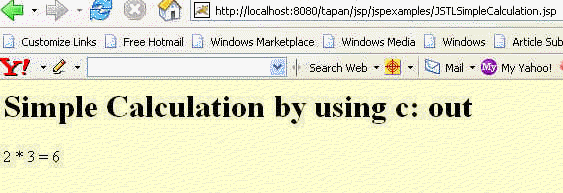Tutorials

# in JSTL

One of the general purpose core library tag is . The main function of the this tag is to display the output to the user.

One of the general purpose core library tag is . The main function of the this tag is to display the output to the user.

# <c: out> in JSTL

One of the general purpose core library tag is <c: out>. The main function of the this tag is to display the output to the user. It works like expression tag in jsp <%= ---%>.

Attributes of the <c: out> core action tag.

1. value: It specify the expression which needs to be evaluated.
2. default: We can use this attribute if the resulting value is null.
3. escapeXml: It checks whether there is any need to convert the &, <, > etc to their character encoding codes.

In this example we have used only one attribute i.e. value. It is a must attribute in the tag. We are just calculating the multiplication of two numbers by using the <c: out> core action tag.

The code of the example is given below:

 <%@ taglib prefix="c" uri="http://java.sun.com/jstl/core" %> Simple Calculation by using c: out

Simple Calculation by using c: out

<%-- Multiplying the numbers 2 * 3 dynamically --%> 2 * 3 =

The output of the program is given below: